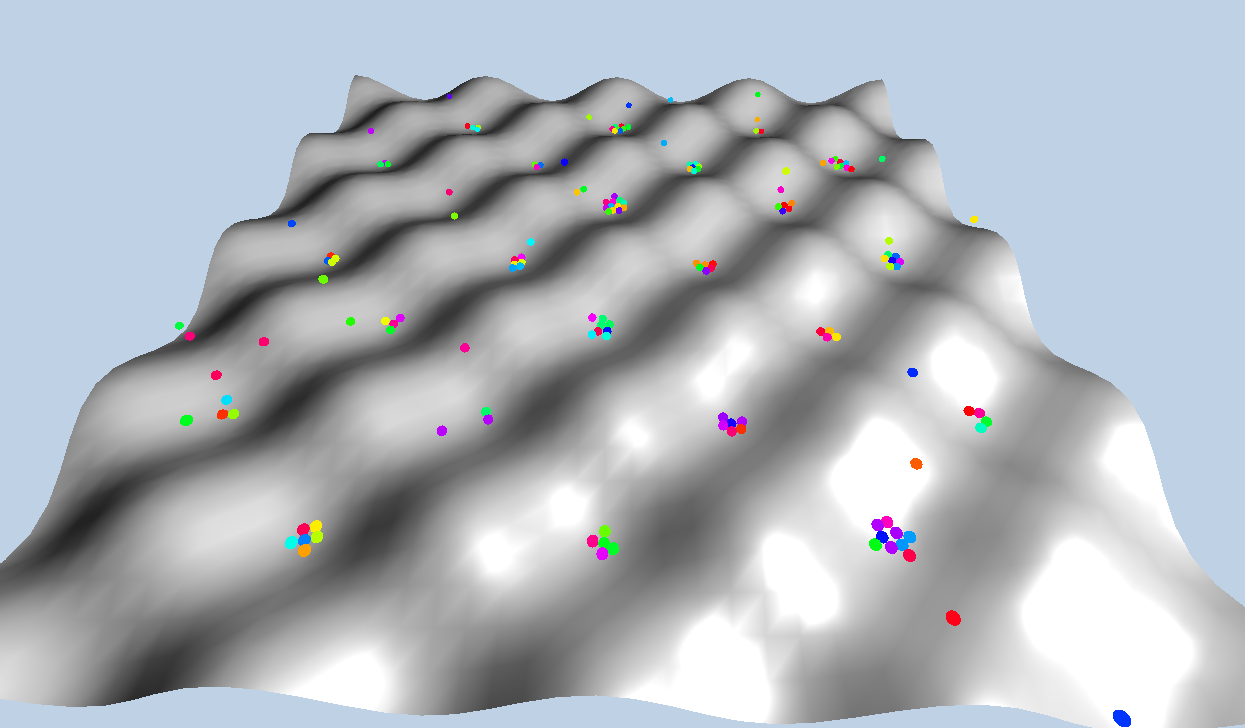﻿ three.js cannon.js物理引擎之Heightfield_其他_开心洋葱网
• 欢迎访问开心洋葱网站，在线教程，推荐使用最新版火狐浏览器和Chrome浏览器访问本网站，欢迎加入开心洋葱` QQ群`
• 为方便开心洋葱网用户，开心洋葱官网已经开启复制功能！
• 欢迎访问开心洋葱网站，手机也能访问哦~欢迎加入开心洋葱多维思维学习平台` QQ群`
• 如果您觉得本站非常有看点，那么赶紧使用Ctrl+D 收藏开心洋葱吧~~~~~~~~~~~~~！
• 由于近期流量激增，小站的ECS没能经的起亲们的访问，本站依然没有盈利，如果各位看如果觉着文字不错，还请看官给小站打个赏~~~~~~~~~~~~~！

# three.js cannon.js物理引擎之Heightfield

1582次浏览## 1. Heightfield的用法

`Heightfield ( data , options )`

data是一个Y值数组，将用于构建地形。options是一个配置项，有三个可配置参数。minValue是数据数组中数据点的最小值。如果未给出，将自动计算。maxValue最大值。elementSize是X方向上数据点之间的世界间距。他还有一些属性和方法请大家自行观看，我就不多说了。

## 2. Heightfield案例

```initCannon() {
//这里是生成高度场的代码
var matrix = [];//创建构造高度场的数组
for (var i = 0; i < size; i++) {
matrix.push([]);
for (var j = 0; j < size; j++) {
//高度由两个余弦函数叠加形成
var height = Math.cos(i / size * Math.PI * 8) * Math.cos(j / size * Math.PI * 8);
matrix[i].push(height)
}
}
var hfShape = new CANNON.Heightfield(matrix, {
elementSize: 1 //数据点的距离设置为1
});
var hfBody = new CANNON.Body({ mass: 0, material: physicsMaterial});
var q = new CANNON.Quaternion();
q.setFromAxisAngle(new CANNON.Vec3(1, 0, 0), - Math.PI / 2);
},
initThree() {
//使用ParametricBufferGeometry配合Heightfield形成网格
var groundGeom = new THREE.ParametricBufferGeometry((u,v,target) => {
var height = Math.cos(u * Math.PI * 8) * Math.cos(v * Math.PI * 8);//这里的方法和生成高度场的方法是一样的，不了解ParametricBufferGeometry几何体的可以参考我之前发的博客。
target.set(u * size - size / 2, height, v * size - size / 2);
}, size, size)
let groundMate = new THREE.MeshPhongMaterial({color: 0x666666, side: THREE.DoubleSide});
groundMesh = new THREE.Mesh(groundGeom, groundMate);